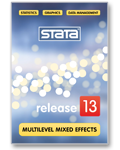»  Home »  Products »  Stata 13 »  Multilevel models

## Multilevel models

### Highlights

• Multilevel estimators
• Continuous outcomes, modeled as
• linear
• log linear
• log gamma
• Binary outcomes, modeled as
• logistic
• probit
• complementary log-log
• Count outcomes, modeled as
• Poisson
• negative binomial
• Categorical outcomes, modeled as
• Ordered outcomes, modeled as
• which is to say,
• generalized linear models (GLM)
• New features
• Nested models (hierarchical)
• Crossed models
• Random intercepts
• Random coefficients (slopes)
• Constraints on variance components
• Cluster–robust SEs to relax distributional assumptions and allow for correlated data
• Posterior mode and mean estimates of random effects

### Show me

You can fit a wide variety of random-intercept and random-slope models.

Let us show you an example with an ordered categorical outcome, random intercepts, and three-level data.

Using a four-level Likert scale, we ran an experiment measuring students' attitudes toward statistics after taking an introductory statistics class. In some of the classes, Stata was used. Other packages were used in the remaining classes. The question is, does exposure to Stata result in a more positive attitude toward statistics?

In the model we fit, we control for use of Stata, each student's average score in previous math courses, and whether either of the student's parents is in a science-related profession.

We will imagine that the fictional data were collected from various courses at various undergraduate schools. School may have an effect, as might class within school.

The results are

. meologit attitude mathscore stata##science || school: || class: Fitting fixed-effects model: Iteration 0: log likelihood = -2212.775 Iteration 1: log likelihood = -2125.509 Iteration 2: log likelihood = -2125.1034 Iteration 3: log likelihood = -2125.1032 Refining starting values: Grid node 0: log likelihood = -2152.1514 Fitting full model: Iteration 0: log likelihood = -2152.1514 (not concave) Iteration 1: log likelihood = -2125.9213 (not concave) Iteration 2: log likelihood = -2120.1861 Iteration 3: log likelihood = -2115.6177 Iteration 4: log likelihood = -2114.5896 Iteration 5: log likelihood = -2114.5881 Iteration 6: log likelihood = -2114.5881 Mixed-effects ologit regression Number of obs = 1600
 No. of Observations per Group Group Variable Groups Minimum Average Maximum school 28 18 57.1 137 class 135 1 11.9 28
Integration method: mvaghermite Integration points = 7 Wald chi2(4) = 124.39 Log likelihood = -2114.5881 Prob > chi2 = 0.0000
 attitude Coef. Std. Err. z P>|z| [95% Conf. Interval] mathscore .4085273 .039616 10.31 0.000 .3308814 .4861731 1.stata .8844369 .2099124 4.21 0.000 .4730161 1.295858 1.science .236448 .2049065 1.15 0.249 -.1651614 .6380575 stata#science 1 1 -.3717699 .2958887 -1.26 0.209 -.951701 .2081612 /cut1 -.0959459 .1688988 -0.57 0.570 -.4269815 .2350896 /cut2 1.177478 .1704946 6.91 0.000 .8433151 1.511642 /cut3 2.383672 .1786736 13.34 0.000 2.033478 2.733865 school var(_cons) .0448735 .0425387 .0069997 .2876749 school>class var(_cons) .1482157 .0637521 .063792 .3443674
LR test vs. ologit regression: chi2(2) = 21.03 Prob > chi2 = 0.0000 Note: LR test is conservative and provided only for reference.

(stata##science is how we introduce a full factorial interaction of stata and school in Stata; see Factor variables and value labels.)

We discover that exposure to Stata does indeed improve students' attitudes toward statistics.

The effect of school is minimal (the variance is small).

Class has a larger effect as revealed by its larger variance, so teachers matter.

Above we showed you an example with random intercepts. We could just as easily have shown you an example with random slopes.

### Show me moreSee the all-new 358-page Multilevel Mixed-Effects Reference Manual.

The manual demonstrates many of the possible models, links, and families, including:

#### Background: What does multilevel mean?

In multilevel data, observations—subjects, for want of a better term—can be divided into groups that have something in common:

1. perhaps the subjects are students, and the groups share having attended the same high school;
2. they are patients that share having been treated at the same hospital; or
3. they are tractors that were manufactured at the same factory.
Whatever it is they share, it may be reasonable to assume that the shared attribute has an effect on the outcome being modeled:
1. Some high schools are better (or worse) than others, so it would be reasonable to assume that the identity of the high school had an effect.
2. The argument is much the same for hospitals when the outcome is subsequent health; some hospitals are better (or worse) than others, at least with respect to particular health problems.
3. For tractors and factories, it would hardly be surprising if tractors from some factories were more reliable than tractors from other factories.
Described above is two-level data:
1. The first level is the student, patient, or tractor.
2. The second level is high school, hospital, or factory.
Stata 13's new multilevel mixed estimation commands handle two-, three-, and higher-level data. With three- and higher-level models, data can be nested or crossed.

See New in Stata 17 to learn about what was added in Stata 17.